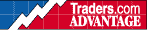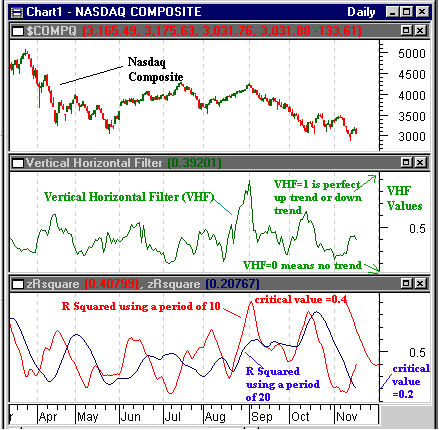HOT TOPICS LIST

INDICATORS LIST

LIST OF TOPICS

# R-Squared and Nasdaq

11/20/00 03:30:27 PM
by Dennis D. Peterson

Is the Nasdaq in a trend or not? R-squared, a by-product of linear regression, is one way to find out. Does it do better than the vertical horizontal filter (VHF), another trend measuring indicator?

Security:   \$compq
Position:   N/A

 When conducting linear regression analysis, one of the by-products you obtain is the measure of how well the linear regression fits the data. A clue to how you might use linear regression to determine if there is a trend or not comes from answering the following question: If all the data were to fit exactly on the regression line what would be the sum of distances from the data to the line? The answer is zero. Linear regression draws a straight line through a series of data points that minimizes the distance between the data and the straight line. In other words, for the Nasdaq, if you draw a straight line through closing daily (or weekly or monthly) data and it turns out that every data point is on the line, then for a linear regression, the sum of the errors is zero. Chande calls this term SSE and SSE = squared [sum (differences between linear regression line and actual data values)].SSE is zero if all of the actual data values are on the linear regression line. Now comes a clever device to see the trend. If a linear regression line is horizontal then it means that data is moving sideways (trendless). But how do you characterize trendless data in terms of linear regression? Suppose you draw a line through the average value of the data. If you are interested in, for example daily data, then a period of time where the data for every day is equal means the average is equal to each daily data point. Chande then created SST as SST=squared [sum (differences between average and actual values)].Obviously if the data is perfectly trendless then SST is zero because the average equals each piece of data. In other words, for a perfectly trendless period the data for every day is the same and the average is therefore equal to any point. In the extreme case, if the data were perfectly vertical then SST will be a BIG number. Also, if you have a perfectly trendless set of data then SSE (zero errors because one line has all of the data on it) equals SST, or SST -SSE is zero. As the trend becomes stronger, SST gets larger, so the formula wants to compare the difference (SST-SSE) to the amount of trend:R-squared = (SST-SSE)/SST.In Metastock the formula for a smoothed r-squared is period:=Input("R squared period",1,100,14);Mov(Pwr(Corr(Cum(1),C,period,0),2),period,S),See the 10/26/00 Traders Advantage posting for the Excel formula of the forecast oscillator. Column G displays the value of r-squared. The higher the value of r-squared the greater the strength of the trend.Figure 1: Nasdaq (top chart), VHF for a 10-day period (middle chart), and the smoothed r-squared for periods of 10 and 20 days (bottom chart). Graphic provided by: MetaStock. Smoothed r-squared is the simple moving average of r-squared and when the two are plotted (Figure 1: bottom chart) you can see that, relative to the Nasdaq (Figure 1: top chart), a 10-day smoothed r-squared is more responsive than the 20-day r-squared, using smoothing periods (moving averages) of 10 and 20 days respectively. Chande proposes critical values to see if the r-squared is statistically significant or not. For an r-squared of 10 this is a critical value of 0.4 and means that with 95% confidence the value of r-squared is significant. For a 20-period r-squared the critical value is 0.2. Remember neither of these give a direction to the trend, just whether one exists or not. The slope of the linear regression line indicates the direction of the trend. A positive slope suggests an uptrend and negative a downtrend. VHF seems to correlate well with the 10-day smoothed r-squared of 10 days. How well? The correlation between the 10-day VHF and the 10-day smoothed r-squared averages between 0.9 and 0.95. They are highly correlated, showing that the two are about even in performance. They both lag, but on balance r-squared does better. So, at this point the Nasdaq is becoming trendless. Prior to this there was a strong downtrend. If the Nasdaq goes into another continuation pattern it means we are likely to go down farther. I don't take exception to those who talk about 2800. I have nothing to show otherwise. From Coppock we are more bearish than we were six months back. In contrast to the NYSE where the number of daily new highs exceeds the number of new lows frequently, the Nasdaq sees very bearish signs, such as today (11/17), when the Nasdaq has 31 new highs and 235 new lows, while the NYSE has 81 new highs and 78 new lows. R-squared and VHF are confirming indicators. Are we misreading candlestick patterns? Is sentiment really bearish? Continuation patterns following strong trends are most likely to break out to continue the trend. Did we have a strong downtrend? Typical of the Nasdaq, a bullish candlestick on 11/14 was followed by spinning top on 11/15, signaling indecision. On 11/16 a bearish candlestick helped cast a bearish shadow. Individual investor sentiment is currently bearish, meaning that the market is oversold, but a bear market can stay oversold. On 9/1 individual investor sentiment was very bullish, which meant overbought, but in a continuation pattern that started with a strong downtrend (see Figure 1: bottom chart of 11/03 posting), it meant the start of a retracement. The turning point was breaking the 3500 support line because we gapped up on 6/2 to a close of 3813 from a close of 3582 on 6/1. This meant that a lot of people who wanted to hang onto profits had stop-losses at that range. When you look at the action at the beginning of October it's not hard to see several stop-losses kicking in, which drove down the market. October second and third were the pivotal dates the Nasdaq went through potential profits made from 6/1/00 and 6/2/00.

Dennis D. Peterson

Market index trading on a daily basis.

 Comments or Questions? Article Usefulness 5 (most useful) 4 3 2 1 (least useful)

Date:�/ /Rank:�4Comment: